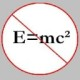## The Dual Isogeny II

Apologies that I haven’t been posting recently. Sage days was this past weekend along with 6 inches of snow in Georgia(read: power outages), the Arizona Winter school in a week and everything else, it’s been very busy.

I’ve also been thinking about the comments on my last post, and how to deal with the dual isogeny in a more comprehensive way. My solution has been to talk about the dual isogeny in terms of maps on$Pic^0(C)$ for$C$ a smooth curve. In the general setting of abelian varieties, the dual isogeny will be a map between the dual abelian variety, a quick set of details on which can be found here or in more detail in the Milne notes on abelian varieties.

Now in the context of elliptic curves, this is nice because we showed earlier that the chord and tangent group law on$E$ is isomorphic to the standard group law on$Pic^0(E)$. Even better, last time we defined the pushforward of an isogeny$f: E_1 \to E_2$ to$f_*:Pic^0(E_1) \to Pic^0(E_2)$ by the map on divisors$\sum_P n_P P \mapsto \sum_P n_P f(P)$. Thus the composite map$E_1 \to Pic^0(E_1) \stackrel{f_*}{\to} Pic^0(E_2) \to E_2$ must be$f$ as for all$P\in E_1$,$P \mapsto P- O_1 \mapsto f(P) - f(O_1) = f(P) - O_2 \mapsto f(P)$.

So we are reduced to looking at maps between$Pic^0$ groups. We define the ramification index of a point$P\in f^{-1}(Q)$  as follows: Since elliptic curves are regular and 1-dimensional, every local ring is a PID. Let$\pi$ be a generator of the unique maximal ideal of the local ring at$Q$.

Example: Consider$\mathbb{P}^1$. The function field of$\mathbb{P}^1$ over a field$k$ is isomorphic to$k(x)$ where$x$ is an abbreviation for the coordinate function$\mathbb{P}^1 \to k, [x:y] \mapsto x/y$. A uniformizer for$[0:1]$ is$x$.  A uniformizer for$[1:0]$ is$1/x = \{[x:y] \mapsto y/x\}$. A uniformizer for$[c:0]$ for$c\in k^\times$ is$x-c = \{[x:y]\mapsto x/y -c = \frac{x-cy}{y}\}$.

This is a rational function on$E_2$, so$f^*\pi$ is a rational function on$E_1$. If$P\in E_1$ is a point mapping to$Q$, we define the ramification index$e_f(P/Q)$ of$f$ at$P$ to be$v_P(f^*\pi)$. Define the pullback$f^*Q$ of a point$Q\in E_2$(and of a divisor by linear extension) by$\sum_{f(P) = Q} e_f(P/Q) P$.  Thus if$D$ is a divisor of$E_2$,$f_*f^*D = \deg(f)D$ because$\deg(f) = \sum_{f(P) = Q} e_f(P/Q)$ for any$Q\in E_2$(this is really a result on the factorization of primes in an extension of rings). Thus by the uniqueness of the dual isogeny,$E_2 \to Pic^0(E_2) \stackrel{f^*}{\to} Pic^0(E_1) \to E_1$ must be the dual isogeny$\hat{f}$.

Now note that$f\circ\hat{f}=[\deg(f)]$ because we already know$\hat{f} \circ f = [\deg(f)]$, so$(f\circ \hat{f}) \circ f = f\circ (\hat{f} \circ f) = f \circ \deg(f)$ and because$f$ is an isogeny, this is$[\deg(f)] \circ f$ so as long as$f$ is  nonconstant, it is surjective, so we know$f\circ \hat{f} = [\deg(f)]$.

Note further that$\widehat{f\circ g} = \hat{g}\circ\hat{f}$ because$(\hat{g}\circ\hat{f})\circ(f\circ g) = \hat{g}\circ [\deg(f)] \circ g = [\deg(f)] \circ (\hat{g} \circ g) = [\deg(f)*\deg(g)]$.

Now we recall that$\deg([m]) = m^2$(consider the complex case), so$\widehat{[m]} = [m]$. Note that$\hat{\hat{}} = $. In general,$\hat{\hat{f}} = f$ because$f\circ\hat{f}$,$= [m] = \widehat{[m]} = \widehat{ f\circ \hat{f}} = \hat{\hat{f}} \circ \hat{f}$ which implies our  result.

Now consider the (coarse) moduli space$X_0(N)$ of pairs$(E,C)$ where$E$ is an elliptic curve and$C$ is a cyclic subgroup of$E$ of order$N$. We can alternately consider this as the space of isogenies with kernel$C$,$\phi: E_1 \to E_2$(consider$E_2 = E_1/C$). This is an example of a modular curve and the dual isogeny then gives an involution$\phi \to \hat{\phi}$ which is called the Atkin-Lehner (or sometimes also the Fricke) involution. There are a surprising number of applications for this including Shih’s theorem, the topic of the public portion of my oral.

If one wants to realize$GL_2(F_p)$ as a Galois group over the rationals, one might consider the covering$X(N) \to X(1)$ and use Hilbert’s irreducibility theorem to bring it down from$\mathbf{Q}(X(1)) = \mathbf{Q}(T)$ to$\mathbf{Q}$. Unfortunately, this is not a regular extension over$\mathbf{Q}$, but one can use the involution to get a twist of$X_0(N)$ whose function field is$\mathbf{Q}(T)$ (there exist$N$ for which this happens) and a corresponding regular extension with Galois group$PSL_2(F_p)$ when$\binom{N}{\overline{p}} = -1$.

Next week another critical piece in that puzzle: The Weil Pairing.

This entry was posted in Abelian Varieties, AG From the Beginning, Curves, Uncategorized. Bookmark the permalink.

### 1 Response to The Dual Isogeny II

1.notedscholar says:

When you put a hyperlink to “more,” you’re not kidding!

NS